## Clock display with Python and Tkinter

On github: https://github.com/aewallin/digiclock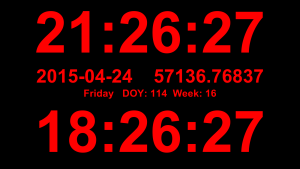A simple clock display with local time, UTC, date (iso8601 format of course!), MJD, day-of-year, and week number. Useful as a permanent info-display in the lab - just make sure your machines are synced with NTP.

This uses two small additions to jdutil:

## Faster allantools with numpy

Update: these slopes are both linear, or O(n), where n is the input data size.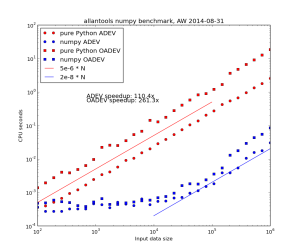However for the worst performing algorithm, MTIE, an O(n*log(n)) algorithm that uses a binary tree to store intermediate results is possible. This algorithm restricts the tau-values to 2**k (with k integer) times the data interval.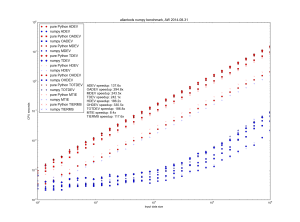Danny Price has made a fantastic contribution to allantools  by taking my previous pure python code (hacked together in January 2014, shown in red) and numpyifying it all (shown in blue)!

For large datasets the speedups are 100-fold or more in most cases!
The speedups are calculated only for datasets larger than 1e5, since python's time.time() doesn't seem suitable for measuring 1 ms or shorter running times.

## Simple Trajectory Generation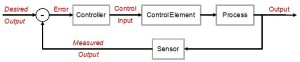The world is full of PID-loops, thermostats, and PLLs. These are all feedback loops where we control a certain output variable through an input variable, with a more or less known physical process (sometimes called "plant") between input and output. The input or "set-point" is the desired output where we'd like the feedback system to keep our output variable.

Let's say we want to change the set-point. Now what's a reasonable way to do that? If our controller can act infinitely fast we could just jump to the new set-point and hope for the best. In real life the controller, plant, and feedback sensor all have limited bandwidth, and it's unreasonable to ask any feedback system to respond to a sudden change in set-point. We need a Trajectory - a smooth (more or less) time-series of set-point values that will take us from the current set-point to the new desired value.

Here are two simple trajectory planners. The first is called 1st order continuous, since the plot of position is continuous. But note that the velocity plot has sudden jumps, and the acceleration & jerk plots have sharp spikes. In a feedback system where acceleration or jerk corresponds to a physical variable with finite bandwidth this will not work well.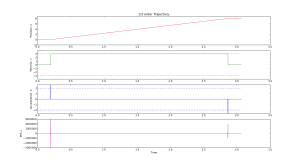We get a smoother trajectory if we also limit the maximum allowable acceleration. This is a 2nd order trajectory since both position and velocity are continuous. The acceleration still has jumps, and the jerk plot shows (smaller) spikes as before.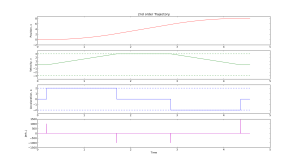Here is the python code that generates these plots:

I'd like to extend this example, so if anyone has simple math+code for a third-order or fourth-order trajectory planner available, please publish it and comment below!

This pattern is for a reply&request situation where one machine asks for data from another machine. The pattern enforces an exchange of messages like this:

REQuester always first sends, then receives.

Requester

The one-way delay here assumes we have machines with synchronized clocks. This might be true to within 1ms or so if both machines are close to the same NTP server.

The two-way delay should be more accurate, since we subtract two time-stamps that both come from the REQuesters system clock. Much better performance can probably be achieved by switching to C or C++.

## ZeroMQ PUB/SUB pattern

I'm playing with zeromq for simple network communication/control. For a nice introduction with diagrams see Nicholas Piel's post "ZeroMQ an introduction"

Here is the PUBlish/SUBscribe patter, which usually consist of one Publisher and one or many Subscribers.

Publisher:

And here is the Subscriber:

## allantools

Python code for calculating Allan deviation and related statistics: https://github.com/aewallin/allantools

Cheat-sheet (see http://en.wikipedia.org/wiki/Colors_of_noise).

 Phase PSD Frequency PSD ADEV 1/f^4, "Black?" 1/f^2, Brownian, random walk sqrt(tau) 1/f^3 ?, "Black?" 1/f Pink constant 1/f^2, Brownian, random walk f^0, White 1/sqrt(tau) f^0, White f^2 Violet 1/tau

Phase PSD is frequency dependence of the the power-spectral-density of the phase noise.
Frequency PSD is the frequency dependence of the power-spectral-density of the frequency noise.
ADEV is the tau-dependence of the Allan deviation.

These can get confusing! Please comment below if I made an error!

## N9 Scratchbox/Harmattan SDK Install

I'm experimenting with compiling programs for the N9.
Go to http://harmattan-dev.nokia.com and get their python script harmattan-sdk-setup.py. Make it executable:
`chmod a+x harmattan-sdk-setup.py`
Run the script as root:
`sudo ./harmattan-sdk-setup.py`
Press "0" for admininstall. This will start a lengthy install. On my machine it started with installing python-qt4. Then scratchbox (89Mb download for X86 and another 89Mb download for ARM). Then comes the SDK itself, one 825 Mb download for the X86-SDK and another 806 Mb download for the ARMEL-SDK.

Now I get:
``` :~/Desktop\$ scratchbox bash: /usr/bin/scratchbox: Permission denied ```
That's because the scratchbox install creates a new user group sbox, and adds your username to this group. We now need to log out of the machine and log in again so that this group is created and your user is added to the group. Now we can run scratchbox. Now, from another terminal on the host-machine, start Xephyr with this:
``` Xephyr :2 -host-cursor -screen 854x480 -dpi 96 -ac +extension Composite & ```
From the scratchbox terminal we can now start meego:
``` meego-sb-session start ```
This gives a little bit of error-messages, but nothing major I guess. And we have a working "phone":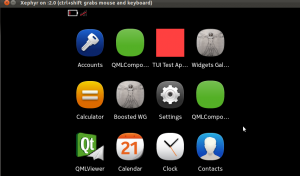If we start Xephyr in portrait mode instead with 480x854x16 it looks a bit better: (should also work by passing '-landscape' flag to meego-sb-session)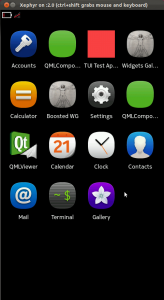The screen where all the open applications are shown looks a bit strange: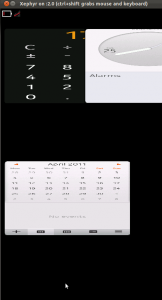This environment should allow coding and compiling in the correct environment on X86, and then cross-compiling on the ARMEL-target and packaging into debs for sending to the device. But on IRC I am told there's an alternative QtSDK toolchain that does the same thing - maybe in a simpler way. To be continued...

## On the density of doubles and floats

With finite precision numbers, if you take a small number eps, there comes a point when x == x + eps. Here's some code that figures out when that happens:

With x around one the resolution limit is roughly at 1e-16 for doubles, and 1e-7 for floats. The top data points show how the relative accuracy stays constant, so at x=1e7 the resolution is only about eps=1 for floats.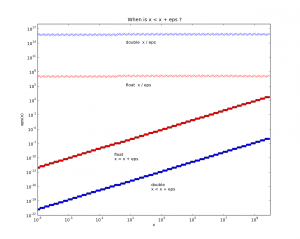Thinking about CAD/CAM related stuff, if you have a fancy top of the line CNC-machine with the longest axis x=1000mm and a theoretical accuracy of one micron, or 0.001mm, then we're at the point where we really should use doubles (which are slower, but how much slower?).

python source for plotting the figure: epsplot.py.tar

## Qt and OpenGL test

It looks like there is no efficient way of updating (adding and removing) triangles in a polydata-surface in VTK, so for the cutting-simulation I am looking at other visualization options. Despite all the tutorials and documentation out there on the interwebs it always takes about two hours to get these "Hello World" examples running...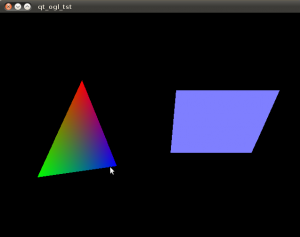Download a zip-file with the source and cmake file: (This compiles and runs on Ubuntu 10.04LTS) qtopengl